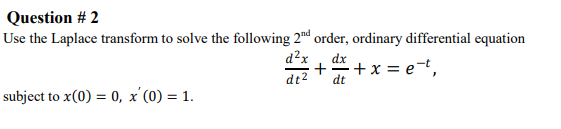### Create an Account

Home / Questions / Question # 2 Use the Laplace transform to solve the following 2nd order, ordinary differen...

# Question # 2 Use the Laplace transform to solve the following 2nd order, ordinary differential equation d2x dx + + x = et, dt2 subject to x(0) = 0, x'(0) = 1. dt

Question # 2 Use the Laplace transform to solve the following 2nd order, ordinary differential equation d2x dx + + x = et, dt2 subject to x(0) = 0, x'(0) = 1. dtMay 17 2021 View more View LessSubscribe To Get Solution